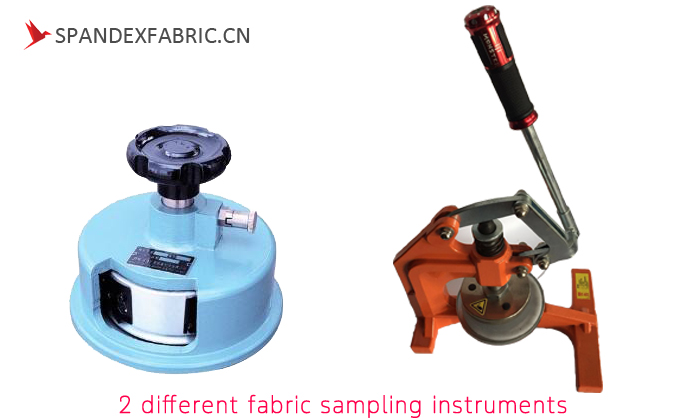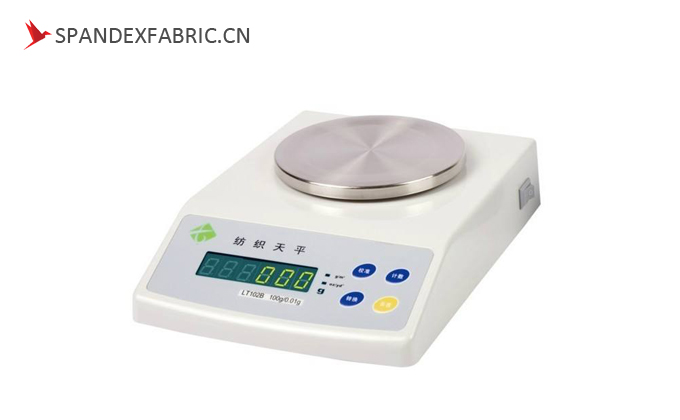We know that it is easy to calculate the weight by fabric GSM scale.

Cut a 100cm2 sample by fabric sampling instrument and weigh it by fabric GSM scale.▲2 different fabric sampling instruments▲fabric GSM scale

But sometimes, we get a sample very small less than 100 cm2, which cannot be cut by fabric sampling instrument.

How can we do it?

Here it is:

`Weight per m2=Test sample scale number × 10000/ Test sample square cm2`

For example

We cut a sample 3 cm×3 cm=9 cm2

we weigh the sample on fabric GSM scale, the result is 0.17

so we can calculate the weight of this fabric is 0.17×10000/9=188.88

considering the inaccuracy in cutting fabric, we can say this fabric weight is 180-200gsm▲Test sample scale number

Why can we use the formula?

Let’s analysis it.

The weight means the weight(g) per 1 m2(=10000 cm2), while the fabric GSM scale test the result in 100 cm2.

so we can get

Scale number/100cm2=fabric weight/10000cm2= Test sample scale number/ Test sample square cm2

So that

Weight per m2=Test sample scale number × 10000/ Test sample square cm2